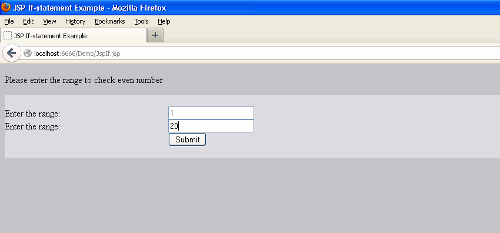# JSP If statement Example

This tutorial will explain about JSP If statement## JSP If statement Example

In this section with the help of example you will learn about the "If" statement in JSP. Control statement is used in every programming languages. The if...else block is started like a simple scriplet but scriplet is closed at every HTML statement. If is used to check for the condition, if it is true then the code inside the if will get executed otherwise else part gets executed. If statement is used for single statement also. Now you are aware of if block, now we are going to use if statement using a simple JSP program.

Example : This example will help you to understand how to use If statement in JSP. We have created one JSP page named "JspIf.jsp", inside this class two text box are created. This program checks for even number using the range input through a text box. In the two text box user have to provide the range under which they want to check the even number. Then another "input.jsp" fetch the value of text box using request.getParameter() and in a loop checking for even number using if statement. Now, here is the code for "JspIf.jsp":

```<%@ page contentType="text/html; charset=iso-8859-1" language="java"%>
<html>
<script type="text/javascript">
function check()
{
var x=document.forms["frm"]["num1"].value;
var y=document.forms["frm"]["num2"].value;
if ((x==null || x=="")||(y==null || y==""))
{
alert("Sorry You have not entered any value");
return false;
}
}
</script>
<title>JSP If-statement Example</title>
<body bgcolor="#C0C0C0">
<form name="frm" method="get" action="input.jsp"
onsubmit="return check()">
<p>Please enter the range to check even number</p>
<font color="#A8E0F7"></font>
bgcolor="#D8D8D8 ">

<tr>
<td width="22%">&nbsp;</td>
<td width="78%">&nbsp;</td>
</tr>
<tr>
<td>Enter the range:</td>
<td><input type="text" name="num1"></td>
</tr>
<tr>
<td>Enter the range:</td>
<td><input type="text" name="num2"></td>
</tr>
<tr>
<td>&nbsp;</td>
<td><input type="submit" name="submit" value="Submit"></td>
</tr>
<tr>
<td>&nbsp;</td>
<td>&nbsp;</td>
</tr>
</table>
</form>
</body>
</html>```

And,  code for "input.jsp" page is as follows:

```
<!DOCTYPE html PUBLIC "-//W3C//DTD HTML 4.01 Transitional//EN" "http://www.w3.org/TR/html4/loose.dtd">
<%@ page contentType="text/html; charset=iso-8859-1" language="java"%>

<html>
<body bgcolor="#C0C0C0">
<%
String a = request.getParameter("num1");
%>
<%
String b = request.getParameter("num2");
%>
<b>Even number program in JSP Using If</b>
<%
int x = Integer.parseInt(a);
int y = Integer.parseInt(b);
for (int i = x; i <= y; i++) {
if ((i % 2) == 0) {
out.print("Even number :" + i);
out.print("<br>");
}
}
%>
</body>
</html>```

When you execute these code, output  will be as follows: